# Lesson 3 6 Implicit Differentiation Objectives Use implicit

• Slides: 8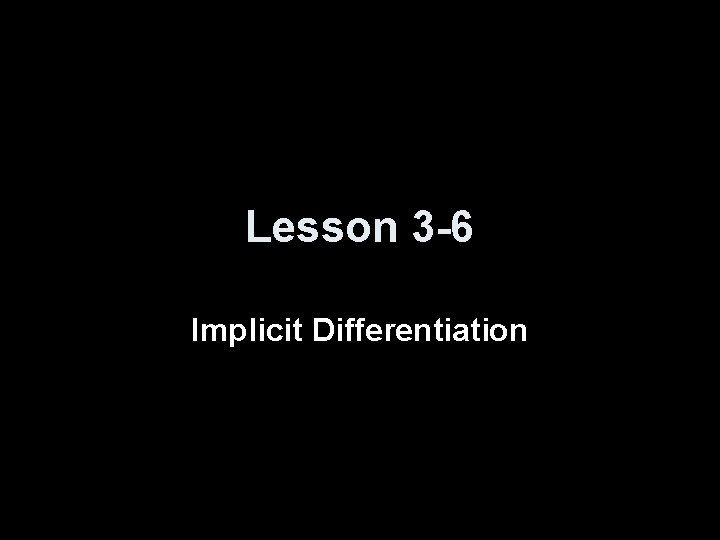Lesson 3 -6 Implicit DifferentiationObjectives • Use implicit differentiation to solve for dy/dx in given equations • Use inverse trig rules to find the derivatives of inverse trig functions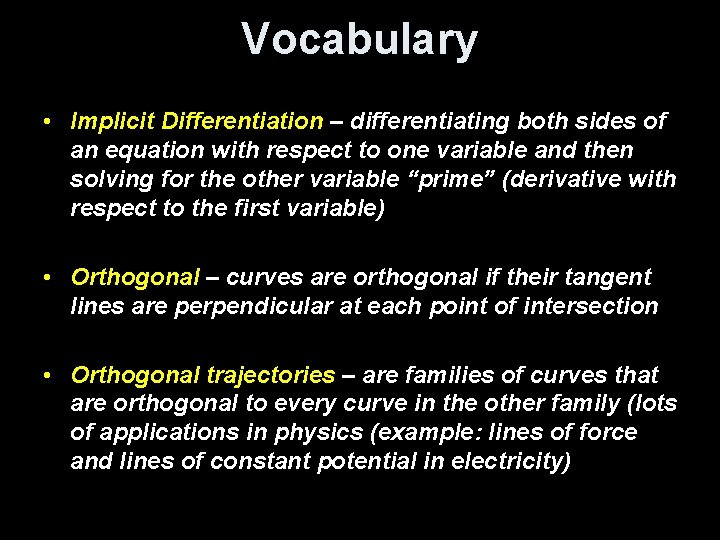Vocabulary • Implicit Differentiation – differentiating both sides of an equation with respect to one variable and then solving for the other variable “prime” (derivative with respect to the first variable) • Orthogonal – curves are orthogonal if their tangent lines are perpendicular at each point of intersection • Orthogonal trajectories – are families of curves that are orthogonal to every curve in the other family (lots of applications in physics (example: lines of force and lines of constant potential in electricity)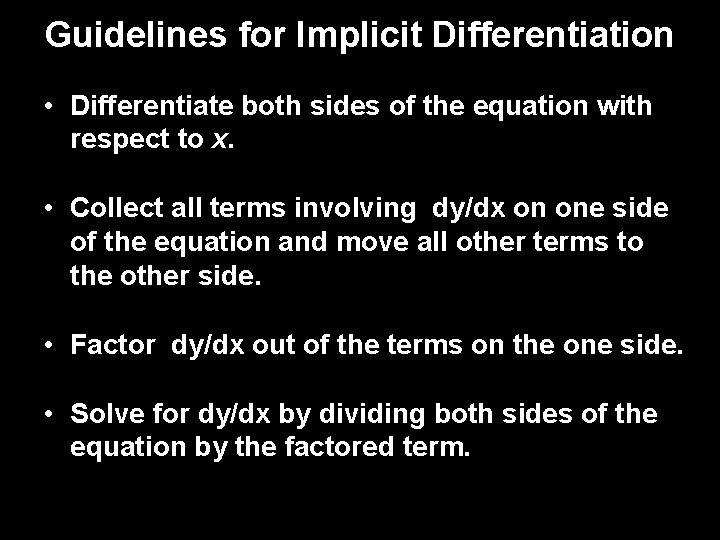Guidelines for Implicit Differentiation • Differentiate both sides of the equation with respect to x. • Collect all terms involving dy/dx on one side of the equation and move all other terms to the other side. • Factor dy/dx out of the terms on the one side. • Solve for dy/dx by dividing both sides of the equation by the factored term.Example 1 Find the derivatives of the following: 1. x = y² - xy + x³ 1 = 2 y (dy/dx) – y – x(dy/dx) + 3 x² 1 + y – 3 x² = (2 y – x) (dy/dx) dy/dx = (1 + y – 3 x²) / (2 y – x) 2. y = (e 4 x²) (cos 6 y) (dy/dx) = 8 x (e 4 x²) (cos 6 y) + (e 4 x²) (-6 sin 6 y)(dy/dx) -8 x (e 4 x²) (cos 6 y) = ((e 4 x²) (-6 sin 6 y) – 1))(dy/dx) -8 x (e 4 x²) (cos 6 y) / ((e 4 x²) (-6 sin 6 y) – 1)) = dy/dx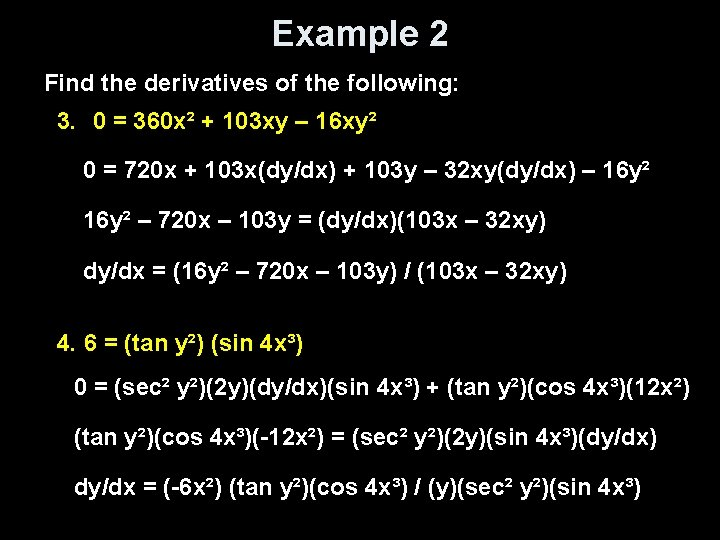Example 2 Find the derivatives of the following: 3. 0 = 360 x² + 103 xy – 16 xy² 0 = 720 x + 103 x(dy/dx) + 103 y – 32 xy(dy/dx) – 16 y² – 720 x – 103 y = (dy/dx)(103 x – 32 xy) dy/dx = (16 y² – 720 x – 103 y) / (103 x – 32 xy) 4. 6 = (tan y²) (sin 4 x³) 0 = (sec² y²)(2 y)(dy/dx)(sin 4 x³) + (tan y²)(cos 4 x³)(12 x²) (tan y²)(cos 4 x³)(-12 x²) = (sec² y²)(2 y)(sin 4 x³)(dy/dx) dy/dx = (-6 x²) (tan y²)(cos 4 x³) / (y)(sec² y²)(sin 4 x³)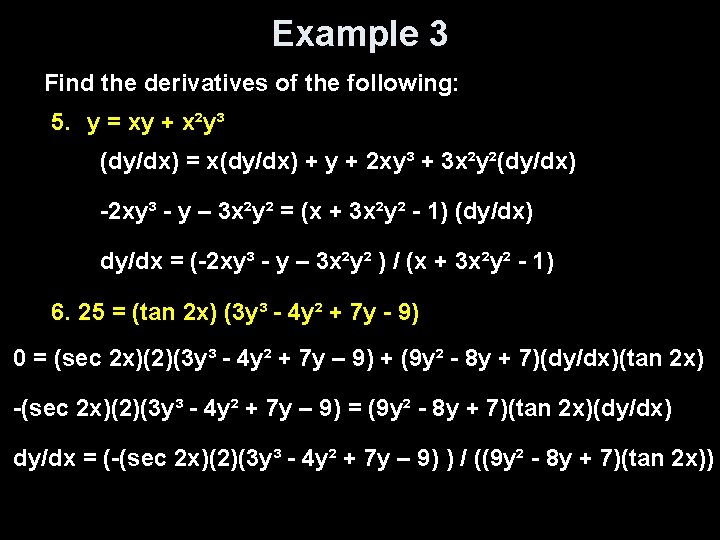Example 3 Find the derivatives of the following: 5. y = xy + x²y³ (dy/dx) = x(dy/dx) + y + 2 xy³ + 3 x²y²(dy/dx) -2 xy³ - y – 3 x²y² = (x + 3 x²y² - 1) (dy/dx) dy/dx = (-2 xy³ - y – 3 x²y² ) / (x + 3 x²y² - 1) 6. 25 = (tan 2 x) (3 y³ - 4 y² + 7 y - 9) 0 = (sec 2 x)(2)(3 y³ - 4 y² + 7 y – 9) + (9 y² - 8 y + 7)(dy/dx)(tan 2 x) -(sec 2 x)(2)(3 y³ - 4 y² + 7 y – 9) = (9 y² - 8 y + 7)(tan 2 x)(dy/dx) dy/dx = (-(sec 2 x)(2)(3 y³ - 4 y² + 7 y – 9) ) / ((9 y² - 8 y + 7)(tan 2 x))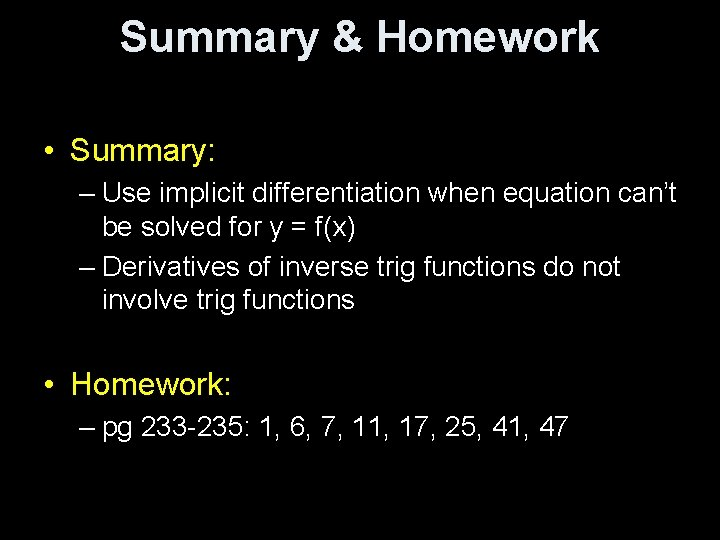Summary & Homework • Summary: – Use implicit differentiation when equation can’t be solved for y = f(x) – Derivatives of inverse trig functions do not involve trig functions • Homework: – pg 233 -235: 1, 6, 7, 11, 17, 25, 41, 47